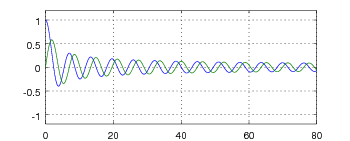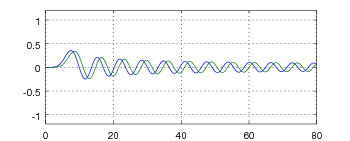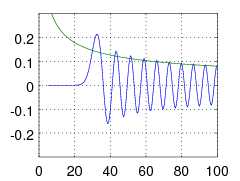There are all sorts of resonances around us, in the world, in our culture, and in our technology. A tidal resonance causes the 55 foot tides in the Bay of Fundy. Mechanical and acoustical resonances and their control are at the center of practically every musical instrument that ever existed. Even our voices and speech are based on controlling the resonances in our throat and mouth. Technology is also a heavy user of resonance. All clocks, radios, televisions, and gps navigating systems use electronic resonators at their very core. Doctors use magnetic resonance imaging or MRI to sense the resonances in atomic nuclei to map the insides of their patients. In spite of the great diversity of resonators, they all share many common properties. In this blog, we will delve into their various aspects. It is hoped that this will serve both the students and professionals who would like to understand more about resonators. I hope all will enjoy the animations.

Origins of Newton's laws of motion

History of mechanical clocks with animations
Understanding a mechanical clock with animations
includes pendulum, balance wheel, and quartz clocks

## Why only J's in a standard Bessel expansion?

In this article we will delve into the question of why some expressions of traveling waves in polar and cylindrical coordinates require the use of the Bessel functions of the second kind in addition to those of the first kind while other situations only require Bessel functions of the first kind.

### Radial and plane wave propagation

For circular traveling waves propagating away from a small source located at the origin, the appropriate solution of the wave equation involves Bessel functions:

in imaginary and real forms respectively. Note that in this formulation Y0 provides for the wave when it has propagated by π/2 radians. We see this in Fig. 1 below. This is similar to the interaction between sine and cosine in a cartesian plane wave:Fig. 1. Bessel functions J0(z) in blue and Y0(z) in green. The horizontal axis indicates the variable z = κr where κ is the wavenumber and r is the radius. Fig 2. Sin(u) in green and cos(u) in blue functions plotted versus u.

Two dimensional simulations of the above wave functions:

 Fig. 3. Two dimensional simulation of radial waves calculated using (1) above. This involved using both kinds of Bessel functions (first and second kinds), i.e. J0 and Y0. Fig 4. Two dimensional simulation of plane waves calculated using (2) above. This involves using both sine and cosine functions.

### Plane wave propagation expressed in polar and cylindrical coordinates

Eq. (1) expresses a radial wave in polar/cylindrical coordinates, while Eq. (2) expresses a plane wave in cartesian coordinates. Expressing each wave type in a different coordinate system is conventional and results in great simplification of the mathematics related to each.

Can we put them both in the same coordinate system to better compare the two types of waves? It turns out we can. There is a standard method for putting a plane wave into polar coordinates. First we insert the polar expression for the cartesian coordinate x:

Inserting (3) into (2) yields:

From here we use a set of Bessel function expansions [Abramowitz and Stegun 9.1.44 and 9.1.45]:

which change (5) into:

Thus, the simple plane wave can be mathematically transformed into an infinite series of Bessel functions in polar coordinates. While not beautiful, at least it is the transformation we sought. As a check we have numerically calculated (6) in Octave and got a result that looks exactly like that shown in Fig. 4 above.

### The question

Why are there no Bessel functions of the second kind (i.e. Y's) in (6)? Don't we need the Y's to complement the J's in a propagating wave as we see in (1) and also in Fig. 3 ?

### Closer look at the J's and Y's

In Eq. (6) above the even orders (orders 0,2,4,6, etc. as in J0, J2, J4, etc.) of Bessel functions are used with one time phase, the  cos ωt  phase. The odd orders of Jn are in the other phase, the  sin ωt  phase. One way to understand this arrangement is to consider pairing up Bessel functions of adjacent orders with each other, so that in each pair there will be one Bessel function of each time phase. In Figs. 5 and 6 we show sets of two typical Bessel function pairings. We see in each pairing the two Bessel functions have phase differences of about π/2 radians just like the Bessel functions J0 and Y0 of Fig. 1 and also between the sine and cosine of Fig. 2. Thus with some complication, adjacent J's can play the same role of providing a shifted wave for our mathematical representation of traveling waves.Fig. 5. J0(z) in blue and J1(z) in green are plotted together to show their π/2 phase difference. Fig 6. J6(z) in blue and J7(z) in green are plotted together to show their π/2 phase difference at larger values of z = κr. For smaller z's the phase shift is less.

So what is the complication of this method?

The problem is due to the cos 2 and cos(2+1) factors in (6). This makes the two adjacent Jn terms have different φ dependence. For example, in the case of J0 and J1 terms, the first has no φ dependence (uniform in all directions) while J1 the term varies as cos φ.

While this difference in φ dependence rules out the use of adjacent Jn's for uniform circular waves as in Fig. 3 this dependence is useful in modeling a plane wave such as shown in Fig. 4. This φ variation allows the modeled wave to be propagating in the positive r direction (positive x direction) to the right of the origin (x > 0) and propagating in the negative r direction (still the positive x direction) in the space to the left of the origin.

Figs. 7 and 8 emphasize this characteristic by using only a very limited number of terms to model the plane wave.

 Fig. 7. Approximation of a plane wave using two terms of the Bessel expansion, Eq. (6), the J0 and Y0 terms. We see that the result is not great but has the correct beginnings of a plane wave focused through the origin. The waves just to the left of the origin are moving towards the origin in the negative r direction but positive x direction. Those just to the right of the origin are moving away from the origin, positive r direction but again in the positive x direction. Note that the origin is located somewhat left of the center of the image (look at the scale to see this). Fig 8. Approximation of a plane wave using four terms of the Bessel expansion, Eq. (6) the J0, J1, Y0 and Y1 terms. We see that the result is significantly better than that in Fig. 7 but is still in need of improvement. Adding more and more terms will bring the approximation closer to modeling a simple plane wave. The higher order terms primarily improve the model at larger radii.

### A look at the asymptotic form of the terms in Equation (6)

For large radii where z = κr ≳ n2 (n is the order) the Bessel functions can be approximated as:

We see the sine/cosine behavior of the Jn/Yn pair, at least at large radii. If we recast the sequential Bessel function Jn+1 as:

then we see the sine/cosine relationship between Jn and Jn+1. The problem with this is that the complete Jn+1 term of Eq. (6) comes with the cos((n+1)φ) factor that doesn't mesh well with that in the Jn term - except if you happen to be able to use it as is done in modeling an inherently cartesian wave, a plane wave. In this case the pairing seems to work just fine.

Spacial resolution of Equation (6)Fig. 9. Bessel function J30(z), in blue. Note how the function is essentially zero until the argument is nearly z ≥ 30. At this point it becomes approximately sinusoidal with an amplitude of approximately √2/πz, shown in green.

Part of the understanding of how a Bessel series mimics a plane wave is to look at the resolution possible with the Bessel series.Fig. 10. Polar plot showing the undulations of each "new" Bessel function at each radius plotted. Thus at the radius where κr ≅ 6  we show cosmφ = cos6φ  as a function of φ in false color (deep red is +1 while deep blue is -1).    The point of this graph is that at every radius, the detailed resolution of the approximation possible with Equation (6) is about constant: that the patch-work seen in this figure shows roughly equal area patches, similar to that possible with Cartesian coordinate waves.

First of all we need to understand some things about the Jn(z) Bessel functions. For one, they are essentially zero until the argument z = κr is greater than or equal to n as demonstrated in the graph of Fig. 9 for J30(z). So for a series approximation at some radius r, only the Bessel functions of order n up through n ≤ κr are non-zero, i.e. important and contribute to the sum.

Secondly, if we look at the largest relevant term in (6) we gain more insight. Fig. 10 shows these largest terms plotted in larger and larger circles. They make up a patch-work quilt, not too dissimilar to that of a Cartesian grid, although somewhat distorted. As we get to larger and larger radii, the area of each patch stays about the same. This figure shows the resolution possible in the Bessel expansion, which we see has similar resolution to a Cartesian grid.